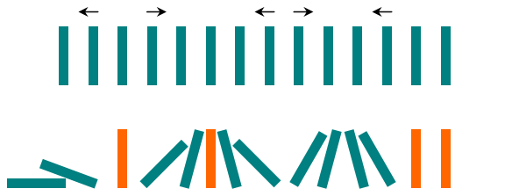# Weekly Contest 85

## 836. Rectangle Overlap [AC]

Leetcode 836

A rectangle is represented as a list [x1, y1, x2, y2], where (x1, y1) are the coordinates of its bottom-left corner, and (x2, y2) are the coordinates of its top-right corner.

Two rectangles overlap if the area of their intersection is positive. To be clear, two rectangles that only touch at the corner or edges do not overlap.

Given two (axis-aligned) rectangles, return whether they overlap.

Example 1:

Example 2:

## 838. Push Dominoes [AC]

Leetcode 838

There are N dominoes in a line, and we place each domino vertically upright.

In the beginning, we simultaneously push some of the dominoes either to the left or to the right.After each second, each domino that is falling to the left pushes the adjacent domino on the left.

Similarly, the dominoes falling to the right push their adjacent dominoes standing on the right.

When a vertical domino has dominoes falling on it from both sides, it stays still due to the balance of the forces.

For the purposes of this question, we will consider that a falling domino expends no additional force to a falling or already fallen domino.

Given a string “S” representing the initial state. S[i] = ‘L’, if the i-th domino has been pushed to the left; S[i] = ‘R’, if the i-th domino has been pushed to the right; S[i] = ‘.’, if the i-th domino has not been pushed.

Return a string representing the final state.

Example 1:

Example 2:

## 837. New 21 Game [unsolved]

Leetcode 837

Alice plays the following game, loosely based on the card game “21”.

Alice starts with 0 points, and draws numbers while she has less than K points. During each draw, she gains an integer number of points randomly from the range [1, W], where W is an integer. Each draw is independent and the outcomes have equal probabilities.

Alice stops drawing numbers when she gets K or more points. What is the probability that she has N or less points?

Example 1:

Example 2:

Example 3:

## 839. Similar String Groups [AC]

Leetcode 839

Two strings X and Y are similar if we can swap two letters (in different positions) of X, so that it equals Y.

For example, “tars” and “rats” are similar (swapping at positions 0 and 2), and “rats” and “arts” are similar, but “star” is not similar to “tars”, “rats”, or “arts”.

Together, these form two connected groups by similarity: {“tars”, “rats”, “arts”} and {“star”}. Notice that “tars” and “arts” are in the same group even though they are not similar. Formally, each group is such that a word is in the group if and only if it is similar to at least one other word in the group.

We are given a list A of strings. Every string in A is an anagram of every other string in A. How many groups are there?

Example 1:

# Weekly Contest 84

## 832. Flipping an Image [AC]

Leetcode 832

Given a binary matrix A, we want to flip the image horizontally, then invert it, and return the resulting image.

To flip an image horizontally means that each row of the image is reversed. For example, flipping [1, 1, 0] horizontally results in [0, 1, 1].

To invert an image means that each 0 is replaced by 1, and each 1 is replaced by 0. For example, inverting [0, 1, 1] results in [1, 0, 0].

Example 1:

Example 2:

## 833. Find And Replace in String [AC]

Leetcode 833

To some string S, we will perform some replacement operations that replace groups of letters with new ones (not necessarily the same size).

Each replacement operation has 3 parameters: a starting index i, a source word x and a target word y. The rule is that if x starts at position i in the original string S, then we will replace that occurrence of x with y. If not, we do nothing.

For example, if we have S = “abcd” and we have some replacement operation i = 2, x = “cd”, y = “ffff”, then because “cd” starts at position 2 in the original string S, we will replace it with “ffff”.

Using another example on S = “abcd”, if we have both the replacement operation i = 0, x = “ab”, y = “eee”, as well as another replacement operation i = 2, x = “ec”, y = “ffff”, this second operation does nothing because in the original string S = ‘c’, which doesn’t match x = ‘e’.

All these operations occur simultaneously. It’s guaranteed that there won’t be any overlap in replacement: for example, S = “abc”, indexes = [0, 1], sources = [“ab”,”bc”] is not a valid test case.

Example 1:

Example 2:

## 835. Image Overlap [AC]

Leetcode 835

Two images A and B are given, represented as binary, square matrices of the same size. (A binary matrix has only 0s and 1s as values.)

We translate one image however we choose (sliding it left, right, up, or down any number of units), and place it on top of the other image. After, the overlap of this translation is the number of positions that have a 1 in both images.

(Note also that a translation does not include any kind of rotation.)

What is the largest possible overlap?

Example 1:

## 834. Sum of Distances in Tree [TLE]

Leetcode 834

An undirected, connected tree with N nodes labelled 0…N-1 and N-1 edges are given.

The ith edge connects nodes edges[i] and edges[i] together.

Return a list ans, where ans[i] is the sum of the distances between node i and all other nodes.

Example 1: